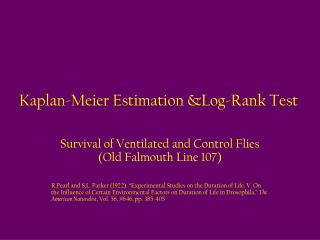Download PresentationKaplan-Meier Estimation &Log-Rank Test

# Kaplan-Meier Estimation &Log-Rank Test - PowerPoint PPT PresentationDownload Presentation## Kaplan-Meier Estimation &Log-Rank Test

- - - - - - - - - - - - - - - - - - - - - - - - - - - E N D - - - - - - - - - - - - - - - - - - - - - - - - - - -
##### Presentation Transcript

1. Kaplan-Meier Estimation &Log-Rank Test Survival of Ventilated and Control Flies (Old Falmouth Line 107) R.Pearl and S.L. Parker (1922). “Experimental Studies on the Duration of Life. V. On the Influence of Certain Environmental Factors on Duration of Life in Drosophila,” The American Naturalist, Vol. 56, #646, pp. 385-405

2. Data Description • 946 Flies are Venilated on Day 1 • 931 Flies are not Ventilated on Day 1 (Controls) • Counts of Survivors and Non-Survivors Reported every 6 Days Subsequently (Days 7,13,…,85) • Goal 1: Estimate the Survival Function for the two groups: S(t) = P(T > t) where T is the (random) Survival time of a fly • Goal 2: Test whether the (population) Survival Functions differ for the 2 Groups

3. Data VentDie and CntlDie represent numbers dying in period just prior to this day

4. Kaplan-Meier Estimation - Notation • For each fly we observe the pair (ti, di) where ti is the ith fly’s time to death or censoring and di is a censoring indicator (1=censored, 0=not censored (actual death)) • Observed failure times: t(1) < … <t(k) • Number of failures at t(i) di • Subjects censored at t(i) treated as if censored between t(i) and t(i+1). Number censored in [t(i),t(i+1))  mi • Subjects at risk Prior to t(i)  ni = (di+mi)+…+(dk+mk) (this removes all who have died or censor prior to t(i)) • Note: For this dataset, there is no censoring (all flies were observed to die)

5. Kaplan-Meier Estimates

6. Kaplan-Meier Estimates Note: For the ventilated flies, 13 died at day 7 (actually between days 1 and 7), out of 946 that were at risk prior to that period: 13/946=.0137. At day 13, 15/933=.0161 was the proportion dying The Survival function at day 7 is obtained as (1-.0137)=.9863. At day 13, the survival function is (1-.0137)(1-.0161) = .9704

7. Log-Rank Test • Used to test whether two (or more) survival functions are equal • Involves obtaining the “expected” number of deaths in (say) the treatment group at time t(i) if the hazard functions for the two groups were equal: • Let n1i and n2i be the numbers at risk just prior to t(i) for the 2 groups, with total at risk  ni = n1i + n2i • Let d1i and d2i be the numbers dying at t(i) for the 2 groups, with total deaths  di = d1i + d2i • Then the expected deaths for group 1 is: e1i=di(n1i/ni) which represents the total deaths at t(i) times the fraction of the total at risk that are in group 1

8. Log-Rank Test

9. Log-Rank Test (Fly Data) Both tests are highly significant